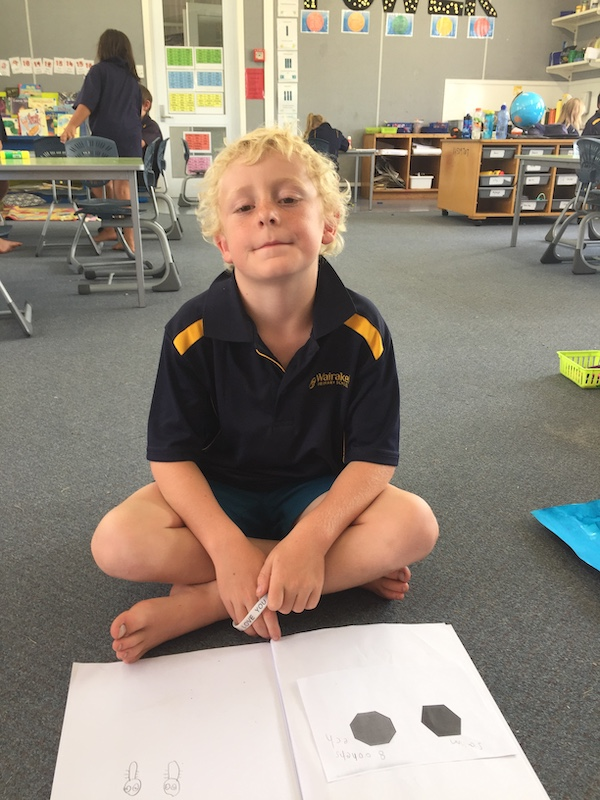# One Is a Snail, Ten is a Crab### One is Snail, Ten is a Crab is a counting feet book and it makes counting really fun.

Room 14 read this book together and then each student got given a lucky a number. The challenge was to make the lucky number by counting the feet of the characters in the story.  For example, a snail has one foot, a person has two, a dog has four feet, an insect has six, a spider has eight, and finally a crab has ten feet. Some of us found there was more than one way to make our lucky number with different characters.

Some of us pretended counters were feet and we made the different animals to work out what combinations of feet would make our number. Some of us drew the different characters we were adding together. Some of us went straight to the numbers and wrote the number sentences that would equal our number.Panias number was 12 she found three different ways to make it

Finally, we wrote the addition number sentence and drew the characters we used to make our lucky numbers.

In math, this sort of activity is called an open-ended question because there can be more than one answer. From doing this activity we also learnt that you can add more than two numbers together. And it does not matter the order we add numbers together, the answer will be the same.Making maths into a visual art display

In the afternoon we turned our math into art and we have made a counting feet display in Room 14.

My number was 12.  I made it from adding two insects.  SethSeth drew two insects to make 12 feet

My number was 10 I made it with a spider and a person.  I also made it with an insect and a dog. It was tricky because I couldn’t use 5 because there are no animals with five legs.  SamNumber 5 was too easy for Sam so he changes his number to 10

My number was 7.  I made it with a dog , a snail and a person AnnabelleAnnabelle and her number sentence

I used a crab, a spider and a snail to make 19.  ZahnZahn and his feet number sentence

I made 22 with two crabs and a person.  Te Omeka

My number was 16 so I made it with 8 + 8 I used two spiders.  Juno

Finally,

Guess what?  We have 44 feet in Room 14. That is:

2 + 2 + 2 + 2 + 2 + 2 + 2 + 2 + 2 + 2 + 2 + 2 + 2 + 2 + 2 + 2 + 2 + 2 + 2 + 2 + 2 + 2 + 2 = 44

Or  22 groups of 2 which is equal to 44

22 X 2 = 44

Next, we are going to work out how many feet are in our houses (including pets) and write the number sentence.Come and see our counting feet display in Room 14

Keywords: maths, open-ended questions, art